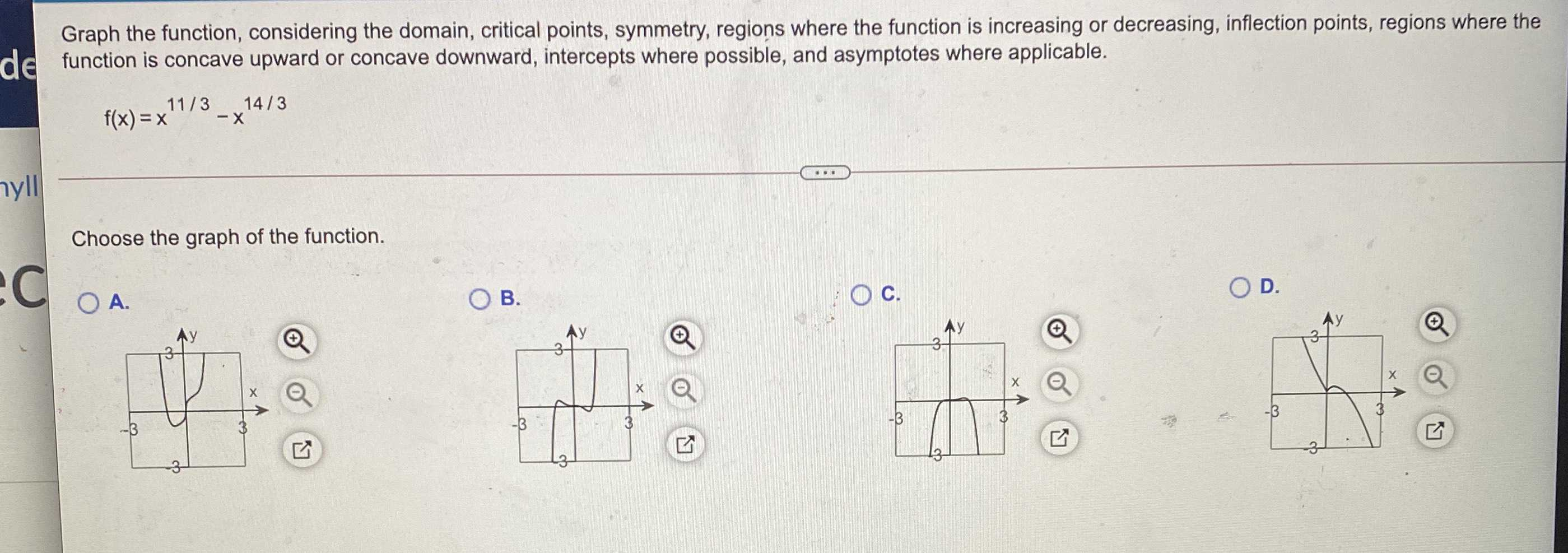### Still have math questions?

Algebra
QuestionGraph the function, considering the domain, critical points, symmetry, regions where the function is increasing or decreasing, inflection points, regions where the function is concave upward or concave downward, intercepts where possible, and asymptotes where applicable.

$$f ( x ) = x ^ { 11 / 3 } - x ^ { 14 / 3 }$$